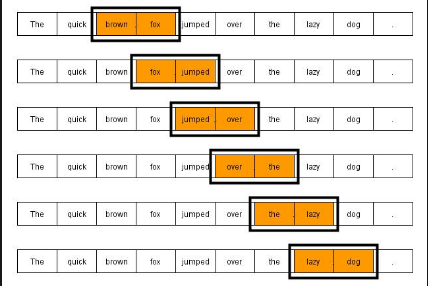# Introduction to n-gram language modelsYou might have heard, that neural language models power a lot of the recent advances in natural language processing. Namely large models like Bert and GPT-2. But there is a fairly old approach to language modeling that is quite successful in a way. I always wanted to play with the, so called n-gram language models. So here’s a post about them.

## What are n-gram language models?

Models that assign probabilities to sequences of words are called language models or LMs. In this post is show you the simplest model that assigns probabilities to sequences of words, the $n$-gram. An $n$-gram is a sequence of $N$ words: a $2$-gram (or bi-gram) is a two-word sequence of words like “This is”, “is a”, “a great”, or “great song” and a $3$-gram (or tri-gram) is a three-word sequence of words like “is a great”, or “a great song”. We’ll see how to use n-gram models to predict the last word of an n-gram given the previous words and thus to create new sequences of words. In a bit of terminological ambiguity, we usually drop the word “model”, and thus the term $n$-gram is used to mean either the word sequence itself or the predictive model that assigns it a probability.

We are using the “German recipes dataset” from kaggle to fit the language model and to generate new recipes from the model.

import numpy as np
import pandas as pd

instructions = data.Instructions


## Tokenize the recipes

Now we use the spacy library to tokenize the recipes and write all tokens to a looong array.

import spacy

nlp = spacy.load('de_core_news_sm', disable=['parser', 'tagger', 'ner'])

tokenized = nlp.tokenizer.pipe(instructions.values)
tokenized = [t.text for doc in tokenized for t in doc]


## Create tri-grams

from collections import Counter, defaultdict
from itertools import tee


We use the tee to return n=3 independent iterators from a single iterable. In this case the word sequence. Then we shift the copied iterables by one and two and zip them to get a “$3$-gram iterator”.

def make_trigrams(iterator):
a, b, c = tee(iterator, 3)
next(b)
next(c)
next(c)
return zip(a, b, c)


Now we can count all $3$-grams appearing in the tokenized word sequence.

trigrams = Counter(make_trigrams(tokenized))


Look at the most common $3$-grams. Obviously, a lot of recipes deal with spicing dishes with salt and pepper, which makes sense.

trigrams.most_common(10)

[(('Salz', 'und', 'Pfeffer'), 4400),
(('Salz', ',', 'Pfeffer'), 2996),
(('mit', 'Salz', 'und'), 2765),
(('.', 'Mit', 'Salz'), 2540),
((',', 'Pfeffer', 'und'), 2169),
(('in', 'einer', 'Pfanne'), 1952),
(('und', 'Pfeffer', 'würzen'), 1831),
(('lassen', '.', 'Die'), 1742),
(('schneiden', '.', 'Die'), 1710),
(('köcheln', 'lassen', '.'), 1669)]


## Build a next-word-lookup

Now we build a look-up from our tri-gram counter. This means, we create a dictionary, that has the first two words of a tri-gram as keys and the value contains the possible last words for that tri-gram with their frequencies.

d = defaultdict(Counter)
for a, b, c in trigrams:
d[a, b][c] += trigrams[a, b, c]


This is how we can look up the next word for “die Möhrensuppe”.

d["die", "Möhrensuppe"]

Counter({'die': 2, 'geben': 7, 'ringsum': 2})


We can use the elements() method of the Counter to get a list of possible words with their frequencies.

list(d["die", "Möhrensuppe"].elements())

['geben',
'geben',
'geben',
'geben',
'geben',
'geben',
'geben',
'die',
'die',
'ringsum',
'ringsum']


## Generate from the model

To generate sequences from the model we simply sample from the above list for a given prefix of a tri-gram and then update the prefix to generate the next word. If we do this multiple times we get a long sequence of words from the model.

import random

def pick(counter):
"""Chooses a random element."""
return random.choice(list(counter.elements()))

prefix = "Eier", "mit"

print(" ".join(prefix), end=' ')
for i in range(100):
suffix = pick(d[prefix])
print(suffix, end=' ')
prefix = prefix, suffix

Eier mit etwas Rotwein ablöschen und kurz mitbraten . Die Zwiebel und die saure Sahne mit dem Öl bei laufendem Mixer einfließen . In einem Topf in 2 El Erdnussöl jetzt die Hälfte der Rosenkohl " Kerne " dazugeben und gut verteilen . Leicht salzen ( wegen der Kinder immer Nudeln und etwas Pul Biber Aci abschmecken . Falls die Oberfläche mit Alufolie verschließen . In einer Pfanne etwas Butter zergehen lassen . Das Eiweiß mit einer Teigrolle zerkleinern . Zitrone auspressen . Die Zwiebeln in Scheiben schneiden und hinzugeben und bei 180 ° C Ober-/Unterhitze stellen und durchziehen lassen und warm


We can see, that the tri-gram model is able to generate quit coherent sentences. But of course there are no proper long-term dependencies. But the results look convincing at first glance.

There are still many more things to do and to cover with $n$-gram language models. We have not implemented anything that deals with out-of-vocabulary words and we have not measured the performance of our model on a test set. Read about smoothing and perplexity to find out more. Or have a look at my post using language models like Bert to do state-of-the-art named entity recognition.